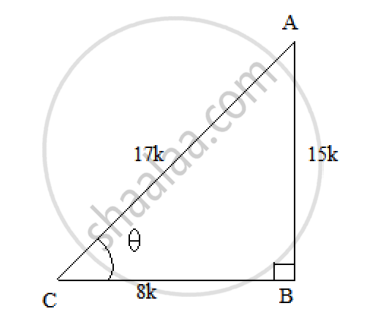# If Sec Theta = 17/8  Verify that ((3-4sin^2theta)/(4 Cos^2theta -3))=((3-tan^2theta)/(1-tan^2theta)) - Mathematics

If sec theta = 17/8  verify that ((3-4sin^2theta)/(4 cos^2theta -3))=((3-tan^2theta)/(1-tan^2theta))

#### Solution

It is given that sec 𝜃=17/8

Let us consider a right ΔABC right angled at B and ∠𝐶 = 𝜃
We know that cos 𝜃 =1/sectheta = 8/17 =(BC)/(AC)So, if BC = 8k, then AC = 17k, where k is a positive number.
Using Pythagoras theorem, we have:
AC^2 = AB^2 + BC^2
⟹ AB^2 = AC^2 − BC^2 = (17K)^2 − (8K)^2
⟹ AB^2 = 289K^2 − 64K^2 = 225K^2
⟹AB = 15k.
Now, tan 𝜃 =(AB)/(BC) =15/8 and sin theta =(AB)/(AC) =(15K)/(17K)=15/17

The given expression is (3-4 sin^2theta)/(4cos^2 theta-3) = (3-tan^2theta)/(1-3tan^2theta)

Substituting the values in the above expression, we get:

LHS=(3-4(15/17)^2)/(4(8/17)^2-3)

=(3-(900/289))/((250/289)-3)

=(867-900)/(256-867)=-33/-611 =33/611

RHS = (3-(15/8)^2)/(1-3(15/8)^2)

= (3-225/64)/(1-675/64)

= (192-255)/(64-675)=-33/-611 = 33/611
∴ LHS = RHS
Hence proved.

Concept: Trigonometric Ratios and Its Reciprocal
Is there an error in this question or solution?

#### APPEARS IN

RS Aggarwal Secondary School Class 10 Maths
Chapter 5 Trigonometric Ratios
Q 23